# PSAT Math : How to find the probability of an outcome

## Example Questions

### Example Question #51 : Probability

A bag contains the following numbers of apples:  12 red delicious, 10 granny smith, and 7 fuji apples.  If an apple is selected at random, what is the probability it is either a granny smith or a fuji apple?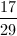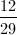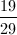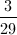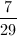Explanation:

Probability = desired outcomes divided by total possible outcomes. Desired outcome can be either fuji or granny smith.

Desired outcomes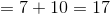Total outcomes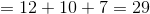### Example Question #52 : Probability

From a class consisting of 15 girls and 12 boys, two students are chosen at random to be class representatives.  What is the probability that neither of the two students chosen is a boy?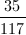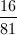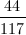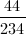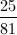Explanation:

To satisfy the question, BOTH students chosen must be girls.

First selection: 15 girls of 27 total students: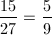Second selection: 14 girls out of 26 total students: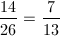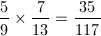### Example Question #52 : How To Find The Probability Of An Outcome

If 2 six-sided dice, each with sides numbered 1-6, are rolled, what is the probability that the sum of the numbers on the face-up sides is equal to 7?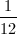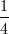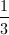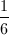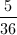Explanation:

Probability = desired outcomes divided by total possible outcomes.

Desired outcomes = 6   (1,6; 2,5; 3,4; 4,3; 5,2; 6;1)

Total outcomes - 36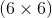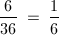### Example Question #61 : Outcomes

If an 8-sided die is rolled 3 times, what is the probability that the same number will be rolled each time?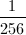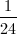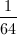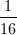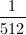Explanation: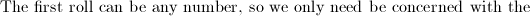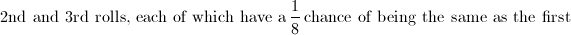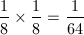Another way to look a it is there are 512 total possible outcomes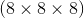and 8 desired outcomes.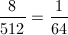### Example Question #61 : Probability

If a two-sided coin is flipped four times, what is the probability of never getting a head?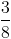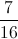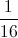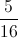Explanation:

The probability of never getting a head is the same as always getting tails. This is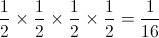.

### Example Question #62 : Outcomes

If a six-sided die is thrown four times, what is the probability of getting a four everytime?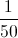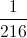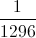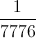Explanation:

The probability is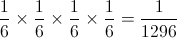.

### Example Question #62 : Probability

If there are 9 marbles in a bag, three white, three blue, and three red, what is the probability of selecting three red marbles at random?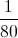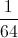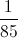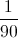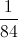Explanation:

The probability of selecting 3 red marbles is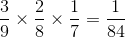.

### Example Question #61 : How To Find The Probability Of An Outcome

There is a box containing 2 red marbles, 7 blue marbles, and an unknown number of green marbles. If the probably of drawing a red marble is 10%, what is the probably that one draws a green marble?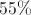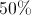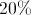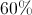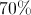Explanation:

Letbe the total number of marbles in the box. Since there's a 10% chance of drawing a red marble, then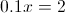implies there are 20 marbles total in the box. Thus, there are only 11 green marbles, resulting in the probability of drawing a green marble being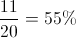### Example Question #61 : Probability

A person rolls a fair 6-sided die twice. What is the probability that the sum of the two rolls is 3?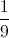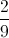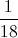Explanation:

In order for the sum to be 3, the two rolls must be 1 and 2. However, it doesn't matter which one comes first. Therefore, there are 2 ways we can acquire the sum being 3. The total number of combination of two die is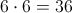.

Thus, the probability of rolling a sum of 3 is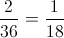.

### Example Question #61 : How To Find The Probability Of An Outcome

A card is drawn randomly from a stardard 52-card deck. What is the probability of drawing a four of hearts?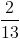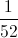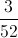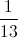Explanation:

The question is asking for the probability of drawing a 4 AND a Heart.

Prob(draw a 4)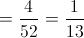.

Prob(draw a Heart)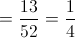.

Then the Prob(4 AND Heart) = Prob(4) * Prob(Heart)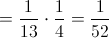You can also solve this question using logic instead of the rules of probability. We know there is only one four of hearts in a deck of cards, so the probability of drawing one must be.

### All PSAT Math Resources